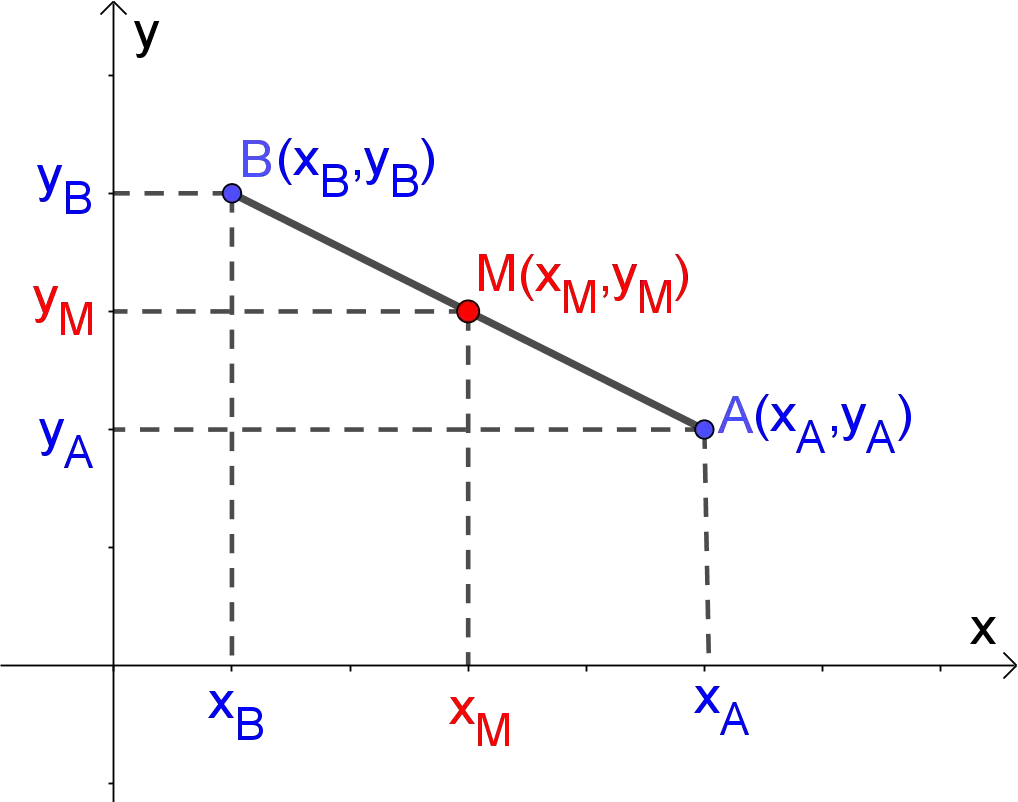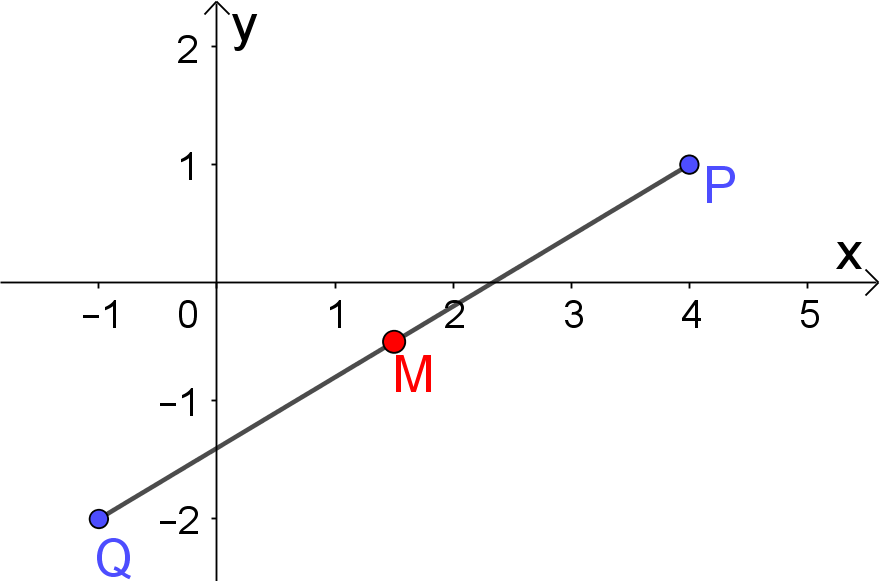# Midpoint Calculator

Coordinates (xA, yA)
Coordinates (xB, yB)
Midpoint (xM, yM)  =  (4, 5)
GENERATE WORK

## Midpoint work with steps

Input Data :
Point 1(x_A, y_A) = (0, 2)
Point 2(x_B, y_B) = (8, 8)

Objective :
Find what is a midpoint of a line segment?

Solution :

 Midpoint (x_M, y_M) =(\frac{x_A + x_B}{2}, \frac{y_A + y_B}{2}) =(\frac{0 + 8}{2}, \frac{2 + 8}{2}) =(\frac{8}{2}, \frac{10}{2})
Midpoint of a line segment (x_M, y_M) = (4, 5)

Midpoint calculator uses coordinates of two points A(x_A,y_A) and B(x_B,y_B) in the two-dimensional Cartesian coordinate plane and find the halfway point between two given points A and B on a line segment. It's an online Geometry tool requires 2 endpoints in the two-dimensional Cartesian coordinate plane. It's an alternate method to finding the midpoint of a line segment without compass and ruler.
It is necessary to follow the next steps:

1. Enter coordinates (x_A,y_A) and (x_B,y_B) of two points A and B in the box. These values must be real numbers or parameters;
2. Press the "GENERATE WORK" button to make the computation;
3. Midpoint calculator will give the coordinates of the midpoint M (x_M , y_M ) of the line segment overline{AB}.
Input: Two ordered number pairs of real numbers. Note that some of coordinate may be variable
Output: An ordered pair of real numbers or variables.

Midpoint Formula:
If we have coordinates of two points A(x_A,y_A) and B(x_B,y_B), then the midpoint of the line segment overline{AB} is determined by the formula
M(x_M,y_M)\equiv M(\frac{x_A + x_B}{2}, \frac{y_A + y_B}{2})## What is the Midpoint?

As we know, a line segment overline{AB} is a part of the line that is bound by two distinct points A and B, which are called the endpoints of the line segment overline{AB}. The point M is the midpoint of the line segment overline{AB} if it is an element of the segment and divides it into two congruent segments, overline{AM}\congoverline{MB}. Each segment between the midpoint M and an endpoint have the equal length. It is often said that the point M bisects the segment overline{AB}. In other words, the midpoint is the center, or middle, of a line segment. Any line segment has a unique midpoint. So, we can find the midpoint of any segment on the coordinate plane by using the mipoint formula.### How to Calculate Midpoint?

The x-coordinate of the midpoint M of the segment overline{AB} is the arithmetic mean of the x-coordinates of the endpoints of the segment overline{AB}. Similarly, the y-coordinate of the midpoint M of the segment overline{AB} is the arithmetic mean of the y-coordinates of the endpoints of the segment overline{AB}.

The work with steps shows the complete step-by-step calculation for how to find the coordinates of center point of line segment having 2 end points A at coordinates (5,8) and B at coordinates (3,2). For any other combinations of endpoints, just supply the coordinates of 2 endpoints and click on the "GENERATE WORK" button. The grade school students may use this midpoint calculator to generate the work, verify the results or do their homework problems efficiently.

Midpoint between 2 Points
(xA, yA) and (xB, yB)Midpoint
(2, 4) and (4, 4)(3, 4)
(0, 2) and (2, 8)(1, 5)
(-4, 5) and (-6, 7)(-5, 6)
(3, -5) and (7, 9)(5, 2)
(1, 0) and (5, 4)(3, 2)
(-7, 5) and (7, 3)(0, 4)
(4, 7) and (2, 9)(3, 8)
(1, 0) and (5, 4)(3, 2)
(2, 0) and (8, 8)(5, 4)
(3, 12) and (9, 15)(6, 13.5)
(6, 5) and (9, 2)(7.5, 3.5)
(1, 7) and (1, 23)(1, 15)
(2, 7) and (6, 3)(4, 5)
(6, 7) and (4, 3)(5, 5)
(1, 7) and (3, 3)(2, 5)
(1, 7) and (3, 2)(2, 4.5)
(8, 5) and (3, 7)(5.5, 6)
(9, 8) and (3, 5)(6, 6.5)
(-1, -6) and (4, 5)(1.5, -0.5)
(-3, -1) and (4, -5)(0.5, -3)
(-4, 4) and (-2, 2)(-3, 3)
(-4, 5) and (-6, 7)(-5, 6)
(-4, 9) and (1, -6)(-1.5, 1.5)
(-5, -7) and (2, -4)(-1.5, -5.5)
(-7, 1) and (3, -5)(-2, -2)

### Real World Problems Using Midpoint

Because an ordered pair of numbers represents coordinates of a point in the two-dimensional Cartesian plane, the midpoint calculator is most often used in analytical geometry. It is also used in other areas of mathematics, especially in the area of complex numbers. For example, a complex number z = a+ib corresponds to the ordered pair of numbers (a, b). It means that the midpoint of the segment connecting z_1 = a + ib and z_2 = c + id in the complex plane is the point (z_1+z_2)/2 with the coordinates:
$$\Big(\frac{a + c}{2}, \frac{b + d}{2}\Big)$$
The midpoint also has applications in physics. The center of mass of an object, is well known as its center of gravity. In other words, is its point of balance. For instance, the midpoint of a ruler is its point of balance. For any line segment, the midpoint is its point of balance, center of mass or center of gravity.

### Practice Problems for Midpoint Calculation

Practice Problem 1:
Belgrade is located at (44.5^o, 20.3^o) and Paris is located at (48.9^o,2.35^o), which represent north latitude and west longitude. If Belgrade and Paris are endpoints of a line segment connecting these towns, find the latitude and longitude of the midpoint of this segment.

Practice Problem 2:
Find coordinates of the midpoint M of \overline{PQ}.The midpoint calculator, formula, step by step calculatio, real world applications and practice problems would be very useful for grade school students (K-12 education) to learn what is midpoint of a line segment in geometry, how to find it and where it can be applicable in real world problems.